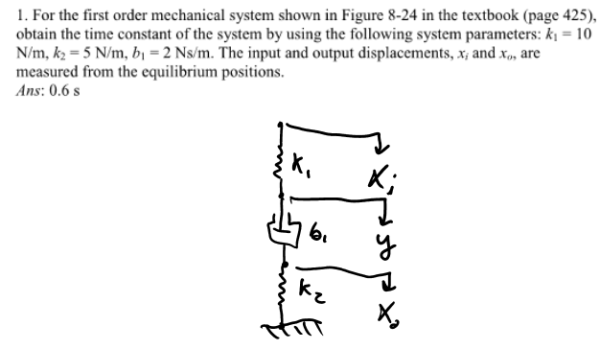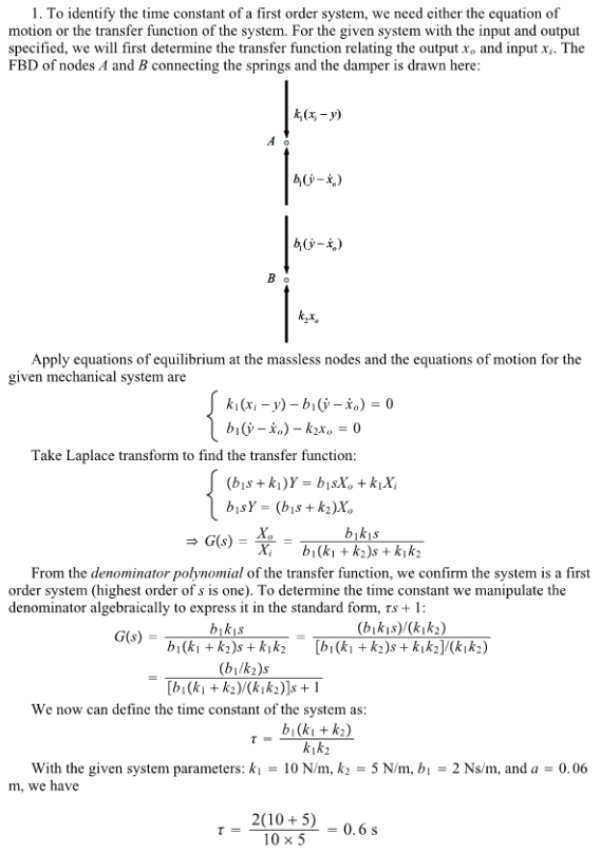For the first order mechanical system shown in the figure in the textbook, obtain the time constant of the system by using the following system parameters k1=10 N/m, k2=5 N/m, b1=2 Ns/m. The input and output displacements, xi and xo, are measured from the equilibrium positions.For the first order mechanical system shown in the figure in the textbook, obtain the time constant of the system by using the following system parameters k1=10 N/m, k2=5 N/m, b1=2 Ns/m. The input and output displacements, xi and xo, are measured from the equilibrium positions.

System Dynamics Page 2 dynamics dynamics dynamics dynamics dynamics dynamics dynamics System dynamics Page 3